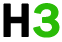C++基础 专题

C++ 命名空间

## 定义命名空间

```namespace namespace_name {
// 代码声明
}
```

```name::code;  // code 可以是变量或函数
```

``````#include <iostream>
using namespace std;

// 第一个命名空间
namespace first_space{
void func(){
cout << "inside first_space" << endl;
}
}
// 第二个命名空间
namespace second_space{
void func(){
cout << "inside second_space" << endl;
}
}
int main ()
{

// 调用第一个命名空间中的函数
first_space::func();

// 调用第二个命名空间中的函数
second_space::func();

return 0;
}``````

```inside first_space
inside second_space
```

## using 指令

``````#include <iostream>
using namespace std;

// 第一个命名空间
namespace first_space{
void func(){
cout << "inside first_space" << endl;
}
}
// 第二个命名空间
namespace second_space{
void func(){
cout << "inside second_space" << endl;
}
}
using namespace first_space;
int main ()
{

// 调用第一个命名空间中的函数
func();

return 0;
}``````

```inside first_space
```

using 指令也可以用来指定命名空间中的特定项目。例如，如果您只打算使用 std 命名空间中的 cout 部分，您可以使用如下的语句：

```using std::cout;
```

``````#include <iostream>
using std::cout;

int main ()
{

cout << "std::endl is used with std!" << std::endl;

return 0;
}``````

```std::endl is used with std!
```

using 指令引入的名称遵循正常的范围规则。名称从使用 using 指令开始是可见的，直到该范围结束。此时，在范围以外定义的同名实体是隐藏的。

## 不连续的命名空间

```namespace namespace_name {
// 代码声明
}
```

## 嵌套的命名空间

```namespace namespace_name1 {
// 代码声明
namespace namespace_name2 {
// 代码声明
}
}
```

```// 访问 namespace_name2 中的成员
using namespace namespace_name1::namespace_name2;

// 访问 namespace:name1 中的成员
using namespace namespace_name1;
```

``````#include <iostream>
using namespace std;

// 第一个命名空间
namespace first_space{
void func(){
cout << "inside first_space" << endl;
}
// 第二个命名空间
namespace second_space{
void func(){
cout << "inside second_space" << endl;
}
}
}
using namespace first_space::second_space;
int main ()
{

// 调用第二个命名空间中的函数
func();

return 0;
}``````

```inside second_space
```